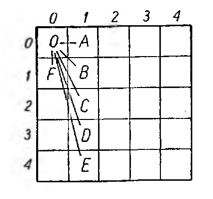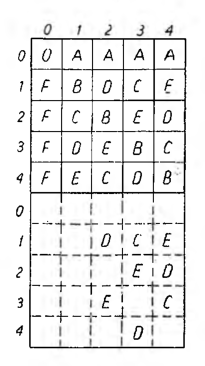# Arithmetic lines part 2

To mark a line step, it is enough to write down two fields closest to each other, through the means of which the line runs, so for example (2,0) i (3,3).

On the basis of this numbering, we can list a further series of fields without looking at the figure, through the means which the line will go through with this step. Enough up to the column number (i.e.. the number in parentheses in the first position) keep adding after 1 = (3 — 2), and keep adding after to the row number 3 = (3 — 0); then we will get such fields:

(2,0), (3,3), (4,6), (5,9), (6,12), (7,15),…

The parts of the lines that go beyond the frame of the first square can always be reduced to analogous steps in that square. For example, let's draw a line (0,0) — (1,2); it will pass through the fields further (2,4), (3,6), (4,8) and so on. Well, a section of this line, namely, her step (3,6) — (4,8), can be reduced to a step (3,1) — (4,3).

If the starting point of the line is the center of the field (0,0), it is wanting to define the fourth field next to the step (1,3), just take the field with the numbers 4 • 1 i 4 • 3, which can be abbreviated:

4 • (1,3), so under these conditions, instead of thrust (0,0), (1,3), (2,6), (3,9), (4,12), (5,15), .. ., you can write a sequence like this:

(0,0), (1,3), 2 -(1,3), 3 -(1,3), 4 .(1,3), 5 .(1.3),…Among the great number of arithmetic lines, which can be carried out from different fields in all directions, we distinguish the so-called main arithmetic lines, marked in the figure below. They all come out of the field (0,0), and their steps are as follows:

step (1,0) that is, OA
" (1,1) ,, OB
" (1,2) „ OC
" (1,3) „ OD
" (1,4) „ OE
oraz krok (0,1) „ OF

The number of main lines for a 25-square square will be 6, it means 5 + 1, by the way: n + 1, if n is the number of squares in a row or column of a square.The main arithmetic lines will pass through the following fields (after bringing their further sections in the second square to the analogous steps in the main square):

Linia OA : (0,0), (1,0), (2,0), (3,0), (4,0)
„ OB : (0,0), (1,1), (2,2), (3,3), (4,4)
„ OC : (0,0), (1,2), (2,4), (3,1), (4,3)
"OD: (0,0), (1,3), (2,1), (3,4), (4,2)
„ OE: (0,0), (1,4), (2,3), (3,2), (4,1)
„ OF : (0,0), (0,1), (0,2), (0,3), (0,4)

We can see this from the list, that each area of ​​the main square will constitute the extreme point of the steps of a certain main line, being just a box (0,0) will be common to all lines, however, in no other field do the main lines meet.

We limit ourselves here to indicating the above few properties of arithmetic lines, needed to understand hypermagic squares, strongly encouraging the Readers to find many other interesting marks on their own. Maybe they will come across real "discoveries."”, and even if they get only things already known, discovered and written in the theory of these lines by their predecessors, they will always benefit from flexing their perceptiveness, sense of orientation and combination.